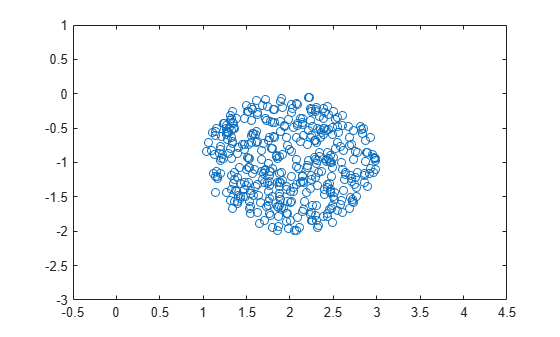## Uncertain Complex Parameters and Matrices

### Uncertain Complex Parameters

The `ucomplex` element is the Control Design Block that represents an uncertain complex number. The value of an uncertain complex number lies in a disc, centered at `NominalValue`, with radius specified by the `Radius` property of the `ucomplex` element. The size of the disc can also be specified by `Percentage`, which means the radius is derived from the absolute value of the `NominalValue`. The properties of `ucomplex` objects are

Properties

Meaning

Class

`Name`

Internal Name

`char`

`NominalValue`

Nominal value of element

`double`

`Mode`

`'Range'```` | ````'Percentage'`

`char`

`Radius`

`double`

`Percentage`

Additive variation (percent of `Radius`)

`double`

`AutoSimplify`

`'off'```` | {````'basic'````} | ````'full'`

`char`

The simplest construction requires only a name and nominal value. Displaying the properties shows that the default `Mode` is `Radius`, and the default radius is 1.

`a = ucomplex('a',2-j)`
```Uncertain complex parameter "a" with nominal value 2-1i and radius 1. ```
`get(a)`
``` NominalValue: 2.0000 - 1.0000i Mode: 'Radius' Radius: 1 Percentage: 44.7214 AutoSimplify: 'basic' Name: 'a' ```

Sample the uncertain complex parameter at 400 values, and plot in the complex plane. Clearly, the samples appear to be from a disc of radius 1, centered in the complex plane at the value 2-j.

```asample = usample(a,400); plot(asample(:),'o'); xlim([-0.5 4.5]); ylim([-3 1]);```### Uncertain Complex Matrices

The uncertain complex matrix class, `ucomplexm`, represents the set of matrices given by the formula

N + WLΔWR

where N, WL, and WR are known matrices, and Δ is any complex matrix with $\stackrel{˙}{\overline{\sigma }}\left(\Delta \right)\le 1$. All properties of a `ucomplexm` are can be accessed with `get` and `set`. The properties are

Properties

Meaning

Class

`Name`

Internal Name

`char`

`NominalValue`

Nominal value of element

`double`

`WL`

Left weight

`double`

`WR`

Right weight

`double`

`AutoSimplify`

`'off'```` | {````'basic'````} | ````'full'`

`char`

#### Uncertain Complex Matrix and Weighting Matrices

Create a 4-by-3 uncertain complex matrix (`ucomplexm`), and view its properties. The simplest construction requires only a name and nominal value.

`m = ucomplexm('m',[1 2 3; 4 5 6; 7 8 9; 10 11 12])`
```Uncertain complex matrix "m" with 4 rows and 3 columns. ```
`get(m)`
``` NominalValue: [4x3 double] WL: [4x4 double] WR: [3x3 double] AutoSimplify: 'basic' Name: 'm' ```

The nominal value is the matrix you supply to `ucomplexm`.

`mnom = m.NominalValue`
```mnom = 4×3 1 2 3 4 5 6 7 8 9 10 11 12 ```

By default, the weighting matrices are the identity. For example, examine the left weighting.

`m.WL`
```ans = 4×4 1 0 0 0 0 1 0 0 0 0 1 0 0 0 0 1 ```

Sample the uncertain matrix, and compare to the nominal value. Note the element-by-element sizes of the difference are roughly equal, indicative of the identity weighting matrices.

```msamp = usample(m); diff = abs(msamp-mnom)```
```diff = 4×3 0.3309 0.0917 0.2881 0.2421 0.3449 0.3917 0.2855 0.2186 0.2915 0.3260 0.2753 0.3816 ```

Change the left and right weighting matrices, making the uncertainty larger as you move down the rows, and across the columns.

```m.WL = diag([0.2 0.4 0.8 1.6]); m.WR = diag([0.1 1 4]);```

Sample the uncertain matrix again, and compare to the nominal value. Note the element-by-element sizes of the difference, and the general trend that the smallest differences are near the (1,1) element, and the largest differences are near the (4,3) element, consistent with the trend in the diagonal weighting matrices.

```msamp = usample(m); diff = abs(msamp-mnom)```
```diff = 4×3 0.0048 0.0526 0.2735 0.0154 0.1012 0.4898 0.0288 0.3334 0.8555 0.0201 0.4632 1.3783 ```